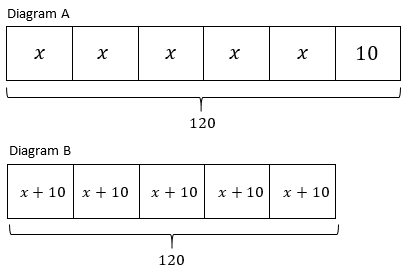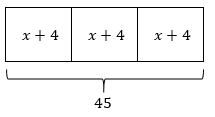# Equations and Inequalities

## Objective

Represent equations in the forms ${px+q=r}$ and ${p(x+q)=r}$ using tape diagrams.

## Common Core Standards

### Core Standards

?

• 7.EE.B.4.A — Solve word problems leading to equations of the form px + q = r and p(x + q) = r, where p, q, and r are specific rational numbers. Solve equations of these forms fluently. Compare an algebraic solution to an arithmetic solution, identifying the sequence of the operations used in each approach. For example, the perimeter of a rectangle is 54 cm. Its length is 6 cm. What is its width?

?

• 6.EE.B.7

## Criteria for Success

?

1. Represent story situations and equations using tape diagrams.
2. Write equations to represent tape diagrams and situations.
3. Write story situations for tape diagrams and equations.
4. Understand how a tape diagram can be helpful to visualize a solution pathway for an equation (MP.1).

## Tips for Teachers

?

Lessons 2 and 3 engage students in representing and solving two-step equations using tape diagrams. In this lesson, students shift between stories, diagrams, and equations, understanding how each connects to the others and helps shed light on the situation. In Lesson 3, students will use the tape diagrams to solve equations.

#### Fishtank Plus

• Problem Set
• Student Handout Editor
• Vocabulary Package

## Anchor Problems

?

### Problem 1

Which tape diagram matches the situation described below? For the other tape diagram, write a story situation that matches it.

Situation:

Five friends go to a fall festival and they each pay an entry fee of $10. The friends each spend the same amount of money on lunch at the festival and don’t spend any other money. Altogether, the friends have spent$120.

Tape Diagrams:### Problem 2

Which equation matches the tape diagram shown below? For the other equation, draw a tape diagram to represent it.

Tape Diagram:Equations:

Equation A:   ${3x+4=45}$

Equation B:   ${3(x+4)=45}$

### Problem 3

For each situation described below, draw a tape diagram and write an equation. Define any variables you use.

Situation A:
A book has 6 chapters in it, each with the same number of pages. The book also has an introduction that is 8 pages long. The whole book is 194 pages long.

Situation B:
Eight baskets have some apples in them, and the same number of apples are in each basket. Six apples are added to each basket to make a total of 144 apples.

## Problem Set

?The following resources include problems and activities aligned to the objective of the lesson that can be used to create your own problem set.

• Given an equation in the form ${px+q=r}$ or ${p(x+q)=r}$, draw a tape diagram and write a story situation.

A family of 5 went to a matinee movie on a Saturday afternoon. The movie tickets for the matinee were a special price for each person. The family spent a combined $25 at the concession stand on drinks and popcorn. Altogether, the family spent$57.50 at the movies.